Disclaimer: This is an example of a student written essay.

Any opinions, findings, conclusions or recommendations expressed in this material are those of the authors and do not necessarily reflect the views of UKEssays.com.

# Solution of a System of Linear Equations for INTELx64

 ✅ Paper Type: Free Essay ✅ Subject: Computer Science ✅ Wordcount: 3182 words ✅ Published: 29th Mar 2018

A multi core hyper-threaded solution of a system of linear equations for INTELx64 architecture

• Richa Singhal

ABSTRACT. A system of linear equations forms a very fundamental principal of linear algebra with very wide spread applications involving fields such as physics, chemistry and even electronics. With systems growing in complexity and demand of ever increasing precision for results it becomes the need of the hour to have methodologies which can solve a large system of such equations to accuracy with fastest performance. On the other hand as frequency scaling is becoming limiting factor to achieve performance improvement of processors modern architectures are deploying multi core approach with features like hyper threading to meet performance requirements. The paper targets solving a system of linear equations for a multi core INTELx64 architecture with hyper threading using standard LU decomposition methodology. This paper also presents a Forward seek LU decomposition approach which gives better performance by effectively utilizing L1 cache of each processor in the multi core architecture. The sample uses as input a matrix of 4000×4000 double precision floating point representation of the system.

1. INTRODUCTION

A system of linear equations is a collection of linear equations of same variable. A system of linear equations forms a very fundamental principal of linear algebra with very wide spread applications involving fields such as physics, chemistry and even electronics. With systems growing in complexity and demand of ever increasing precision for results it becomes the need of the hour to have methodologies which can solve a large system of such equations to accuracy with fastest performance. On the other hand as frequency scaling is becoming limiting factor to achieve performance improvement. With increasing clock frequency the power consumption goes up

P = C x V2 x F

P is power consumption

V is voltage

F is frequency

It was because of this factor only that INTEL had to cancel its Tejas and Jayhawk processors. A newer approach is to deploy multiple cores which are capable to parallel process mutually exclusive tasks of a job to achieve the requisite performance improvement. Hyper threading is another method which makes a single core appears as two by using some additional registers. Having said that it requires that traditional algorithms which are sequential in nature to be reworked and factorized so that they can efficiently utilize the processing power offered by these architectures.

If you need assistance with writing your essay, our professional essay writing service is here to help!

This paper aims to provide an implementation for standard LU decomposition method used to solve system of linear equations adopting a forward seek methodology to efficiently solve a system of double precision system of linear equations with 4000 variable set. The proposed solution addresses all aspects of problem solving starting from file I/O to read the input system of equations to actually solving the system to generate required output using multi core techniques. The solution assumes that the input problem has one and only one unique solution possible.

2. CHALLENGES

The primary challenge is to rework the sequential LU decomposition method so that the revised framework can be decomposed into a set of independent problems which can be solved independently as far as possible. Then use this LU decomposition output and apply standard techniques of forward and backward substitution each again using multi core techniques to reach the final output.

Another challenge associated is cache management. Since a set of 4000 floating point variable will take a memory approximately 32KB of memory and there will 4000 different equations put up together, hence efficiently managing all data in cache becomes a challenge. A forward seek methodology was used in LU decomposition which tries to keep the relevant data at L1 cache before it is required to be processed. It also tries to maximise operations on set of data once it is in cache so that cache misses are minimum.

3. IMPACT

With a 40 core INTEXx64 machine with hyper threading the proposed method could achieve an acceleration of ~72X in performance as compared to a standard sequential implementation.

4. STATE OF THE ART

The proposed solution uses state of the art programming techniques available for multithreaded architecture. It also uses INTEX ADVANCED VECTOR SET (AVX) intrinsic instruction set to achieve maximum hyper threading. Native POSIX threads were used for the purpose. Efficient disk IO was made possible by mapping input vector file to RAM directly using mmap.

5. PROPOSED SOLUTION

A system of linear equations representing CURRENT / VOLTAGE relationship for a set of resistances is defined as

[R][I] = [V]

Steps to solve this can be illustrated as

1. Decompose [R] into [L] and [U]
2. Solve [L][Z] = [V] for [Z]
3. Solve [U][I] = [Z] for [I]

Resistance matrix is modelled as an array 4000×4000 of double precision floating type elements. The memory address being 16 byte aligned so that RAM access speeds up for read and write operations.

FLOAT RES[MATRIX_SIZE*MATRIX_SIZE]

__attribute__((aligned(0x1000)));

Voltage matrix is modelled as an array 4000×1 of double precision floating type elements. The memory address being 16 byte aligned so that RAM access speeds up for read and write operations.

FLOAT V [MATRIX_SIZE] _attribute__ ((aligned(0x1000)));

1. LU Decomposition

To solve the basic model of parallel LU decomposition as suggested above was adopted. Here as we move along the diagonal of the main matrix we calculate the factor values for Lower triangular matrix. Simultaneously each row operation updates elements for upper triangular matrix.

1. Basic routine to do row operation

This routine is the innermost level routine which updates the rows which will eventually determine the upper triangular matrix.

For each element of row there is one subtraction and one multiplication operation (highlighted).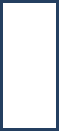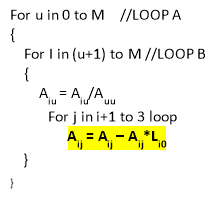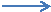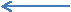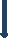U00 U01 U02 U03 L10 U11 U12 U13 L20 L21 U22 U23 L30 L31 L32 U33

LOOP B designates row major operation, while LOOP A designates column major operation.

Basic Algorithm

SUB LUDECOM (A, N)

DO K = 1, n – 1

DO I = K+1, N

Ai, k = Ai, k / Ak, j

DO j = K + 1, N

Ai, j = Ai, j – Ai, k * Ak, j

END DO

END DO

END DO

END LUDECOM

Each row major operation (LOOP B) iteration can be independently executed on a separate core. This was achieved by using POSIX threads which were non-blocking in nature. Because of mutual exclusion over the set of data MUTEX locks are not required provided we keep the column major operation (LOOP A) sequential.

Also for 2 consecutive elements in one row operation 2 subtraction and 2 multiplication operations are done. These 2 operations each are done in single step using Single Instruction Multiple Data instructions (Hyper threading)

Multi core Algorithm

SUB LUDECOM_BLOCK (A, K, BLOCK_START, BLOCK_END)

DO I = BLOCK_START, BLOCK_END

Ai, K = Ai, K / AK, K

DO j = K + 1, N

Ai, j = Ai, j – Ai, K * Ak, K

END DO

END DO

END LUDECOM_BLOCK

SUB LUDECOM (A, N)

DO K = 1, N – 1 BLOCK_SIZE = (N – K) / MAX_THREADS

LUDECOMPOSITION_BLOCK (A,

K,

)

ENDWHILE

END DO

END LUDECOM

1. Forward substitution

Once LU decomposition is done, forward substitution gives matrix [Z]. Here again Single Instruction Multiple Data instructions are used

[L][Z] = [V] for [Z]

1. Backward substitution

After forward substitution final step of backward substitution gives current matrix [I]

[U][I] = [Z] for [I]

Here again Single Instruction Multiple Data instructions are used

5. CACHE IMPROVEMENTS

On profiling it is observed that the core processing in above solution happens to be LU decomposition. However if we create threads equal in number to available cores the result was improving but not in same proportion to the number of cores. A VALGRIND analysis of cache performance reveals that because of large size of matrix each row operation was suffering a performance hit due to cache misses happening.

Our academic experts are ready and waiting to assist with any writing project you may have. From simple essay plans, through to full dissertations, you can guarantee we have a service perfectly matched to your needs.

If we observe above solution it could be observed any jth is processed for (j – 1) columns. So (j – 1) threads are forked for each iteration of column major operation (LOOP A). The data to be processed refers to same memory location but by the time next operation or thread is forked for the same row the corresponding memory data had been pushed out of lower level caches. Thus cache miss happens.

To solve this we adopted a forward seek approach wherein we first pre-process a set of columns sequentially thus enabling more operations on a row to be performed in the same thread. Now the data happens to be at lower level cache as we do not have to wait for another thread to process the same row.

Multi core Algorithm with forward seek operation

SUB LUDECOM_BLOCK_SEEK (A, K, S, BLOCK_START, BLOCK_END)

DO I = BLOCK_START, BLOCK_END

DO U = 1, S

M = K + U -1

Ai, M = Ai, M / AM, j

DO j = K + M + 1, N

Ai, j = Ai, j – Ai, M * AK, M

END DO

END DO

END DO

END LUDECOM_BLOCK

SUB LUDECOM (A, N)

K = 1

WHILE (K <= N)

//Forward seek

DO J = K, K + F_SEEK

LU_DECOM_BLOCK_SEEK (A, J, 0, J, J+F_SEEK)

END DO

//Multi core

K = K + F_SEEK

DO L = 1, N – 1 BLOCK_SIZE = (N – L) / MAX_THREADS

LUDECOMPOSITION_BLOCK (A,

L,

F_SEEK,

)

ENDWHILE

END DO

END WHILE

END LUDECOM

1. CONCLUSION

Results

For purpose of computation a sample array of double precision floating point matrix of size 4000×4000 was taken. Performance numbers were generated on an 8 core INTEL architecture machine.

 Experiment Result Simple linear program 200 Seconds Program with 8 threads (2 Hyper threads in each thread) ~12 Seconds (APPROX. 16x faster) Program with 72 threads on NEHALAM (64 cores) with forward seek ~1 second

TABLE 4.i

A programmer that writes implicitly parallel code does not need to worry about task division or process communication, focusing instead in the problem that his or her program is intended to solve. Implicit parallelism generally facilitates the design of parallel programs and therefore results in a substantial improvement of programmer productivity.

Many of the constructs necessary to support this also add simplicity or clarity even in the absence of actual parallelism. The example above, of List comprehension in the sin() function, is a useful feature in of itself. By using implicit parallelism, languages effectively have to provide such useful constructs to users simply to support required functionality (a language without a decent for loop, for example, is one few programmers will use).

Languages with implicit parallelism reduce the control that the programmer has over the parallel execution of the program, resulting sometimes in less-than-optimal solution The makers of the Oz programming language also note that their early experiments with implicit parallelism showed that implicit parallelism made debugging difficult and object models unnecessarily awkward.

A larger issue is that every program has some parallel and some serial logic. Binary I/O, for example, requires support for such serial operations as Write() and Seek(). If implicit parallelism is desired, this creates a new requirement for constructs and keywords to support code that cannot be threaded or distributed.

REFERENCES

1. Gottlieb, Allan; Almasi, George S. (1989).Highly parallel computing. Redwood City, Calif.: Benjamin/Cummings.ISBN0-8053-0177-1.
2. S.V. Adve et al. (November 2008).“Parallel Computing Research at Illinois: The UPCRC Agenda”(PDF). Parallel@Illinois, University of Illinois at Urbana-Champaign. “The main techniques for these performance benefits– increased clock frequency and smarter but increasingly complex architectures– are now hitting the so-called power wall. The computer industry has accepted that future performance increases must largely come from increasing the number of processors (or cores) on a die, rather than making a single core go faster.”
3. Asanovic et al. Old [conventional wisdom]: Power is free, but transistors are expensive. New [conventional wisdom] is [that] power is expensive, but transistors are “free”
4. Bunch, James R.;Hopcroft, John(1974), “Triangular factorization and inversion by fast matrix multiplication”,Mathematics of Computation28: 231–236,doi:10.2307/2005828,ISSN0025-5718.
5. Cormen, Thomas H.;Leiserson, Charles E.;Rivest, Ronald L.;Stein, Clifford(2001),Introduction to Algorithms, MIT Press and McGraw-Hill,ISBN978-0-262-03293-3.
6. Golub, Gene H.;Van Loan, Charles F.(1996),Matrix Computations(3rd ed.), Baltimore: Johns Hopkins,ISBN978-0-8018-5414-9.

View all

## DMCA / Removal Request

If you are the original writer of this essay and no longer wish to have your work published on UKEssays.com then please:

Related Services

Prices from

AED557

Approximate costs for:

• 1000 words
• 7 day deliveryHumanity University

Dedicated to your worth and value as a human being!

Related Lectures